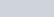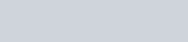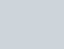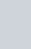From a well shuffled pack of cards, a card is drawn at random.
Question:

From a well shuffled pack of cards, a card is drawn at random. Find the probability of getting a black queen.

Solution:

GIVEN: One card is drawn from a well shuffled deck of 52 playing cards

TO FIND: Probability of getting a black queen

Total number of cards are52.

Cards which are black queen are 1 from 2 black suits

Total number of black queen cards isWe know that PROBABILITY =Hence probability of getting an black queen=Hence probability of getting an black queen =